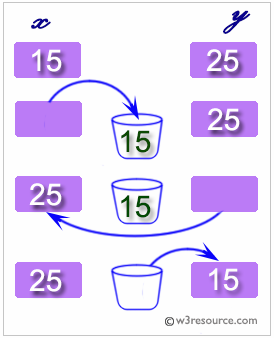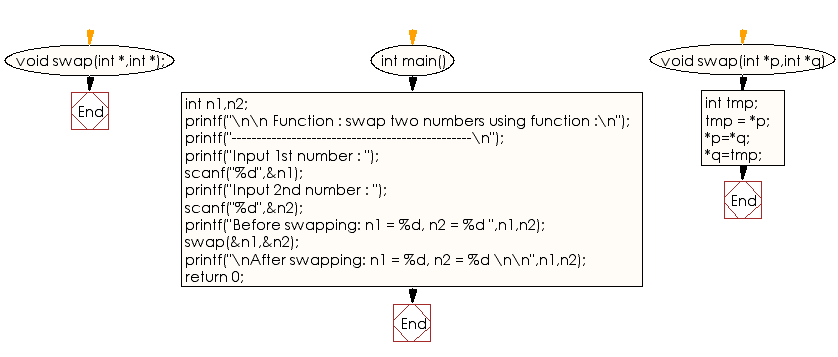﻿ C Program: Swap two numbers using the function - w3resource

# C Exercises: Swap two numbers using the function

## C Function : Exercise-3 with Solution

Write a program in C to swap two numbers using a function.

C programming: swapping two variables

Swapping two variables refers to mutually exchanging the values of the variables. Generally, this is done with the data in memory.

The simplest method to swap two variables is to use a third temporary variable :

```define swap(a, b)
temp := a
a := b
b := temp
```

Pictorial Presentation:Sample Solution:

C Code:

``````#include<stdio.h>

void swap(int *,int *);
int main()
{

int n1,n2;
printf("\n\n Function : swap two numbers using function :\n");
printf("------------------------------------------------\n");
printf("Input 1st number : ");
scanf("%d",&n1);
printf("Input 2nd number : ");
scanf("%d",&n2);

printf("Before swapping: n1 = %d, n2 = %d ",n1,n2);
//pass the address of both variables to the function.
swap(&n1,&n2);

printf("\nAfter swapping: n1 = %d, n2 = %d \n\n",n1,n2);
return 0;
}

void swap(int *p,int *q)
{
//p=&n1 so p store the address of n1, so *p store the value of n1
//q=&n2 so q store the address of n2, so *q store the value of n2

int tmp;
tmp = *p; // tmp store the value of n1
*p=*q;    // *p store the value of *q that is value of n2
*q=tmp;   // *q store the value of tmp that is the value of n1
}
```
```

Sample Output:

``` Function : swap two numbers using function :
------------------------------------------------
Input 1st number : 2
Input 2nd number : 4
Before swapping: n1 = 2, n2 = 4
After swapping: n1 = 4, n2 = 2
```

Explanation:

```void swap(int * p, int * q) {
//p=&n1 so p store the address of n1, so *p store the value of n1
//q=&n2 so q store the address of n2, so *q store the value of n2
int tmp;
tmp = * p; // tmp store the value of n1
* p = * q; // *p store the value of *q that is value of n2
* q = tmp; // *q store the value of tmp that is the value of n1
}
```

The above function ‘swap’ takes two integer pointers as arguments, p and q. It swaps the values stored at the memory locations pointed to by 'p' and 'q'. To do this, it first creates a temporary integer variable named 'tmp' and sets its value to the value stored at the memory location pointed to by 'p'. It then sets the value stored at the memory location pointed to by 'p' to be the value stored at the memory location pointed to by 'q'. Finally, it sets the value stored at the memory location pointed to by 'q' to be the value stored in tmp.

Time complexity and space complexity:

The time complexity of this function 'swap' is O(1), as the computation it performs is constant and independent of the input size.

The space complexity of this function is also O(1), as it only uses a fixed amount of memory to store the temporary integer variable 'tmp' and the two integer pointers 'p' and 'q'.

Flowchart:C Programming Code Editor:

What is the difficulty level of this exercise?

Test your Programming skills with w3resource's quiz.

﻿